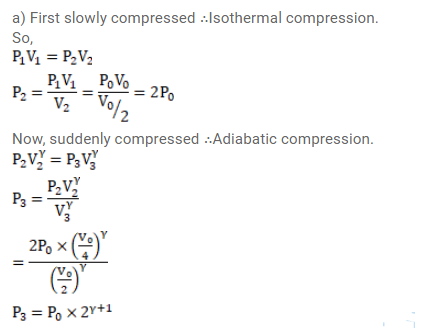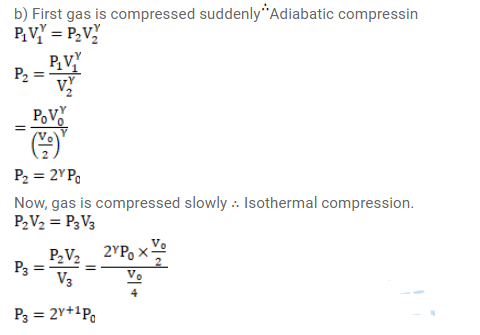# The initial pressure and volume of a given mass of`
Question:

The initial pressure and volume of a given mass of a gas $\left(C_{p} / C_{v}=\gamma\right)$ are $p_{0}$ and $V_{0}$. The gas can exchange heat with the surrounding. (a) It is slowly compressed to $\mathrm{V}_{0} / 4$. Find the final pressure. (b) If the gas is suddenly compressed from the volume $\mathrm{V}_{0}$ to $\mathrm{V}_{0} / 2$ and then slowly compressed to $\mathrm{V}_{0} / 4$, what will be the final pressure?

Solution: